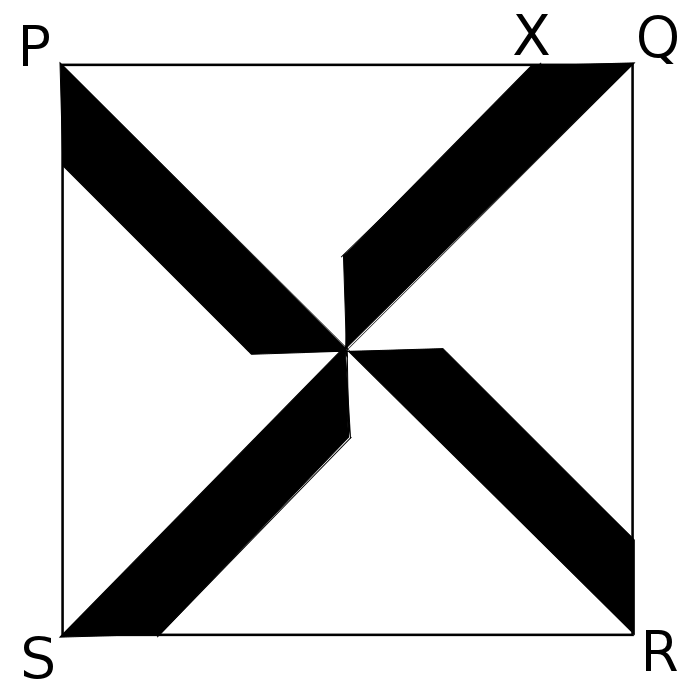You may also likeBuilding Tetrahedra

Can you make a tetrahedron whose faces all have the same perimeter?A 1 metre cube has one face on the ground and one face against a wall. A 4 metre ladder leans against the wall and just touches the cube. How high is the top of the ladder above the ground?Four rods are hinged at their ends to form a convex quadrilateral. Investigate the different shapes that the quadrilateral can take. Be patient this problem may be slow to load.

Quarters

Age 14 to 16 ShortChallenge LevelFour congruent isoceles trapeziums are placed so that their longer parallel sides form the diagonals of a square PQRS, as shown. The point X divides PQ in the ratio 3:1. What fraction of the square is shaded?

If you liked this problem, here is an NRICH task that challenges you to use similar mathematical ideas.

This problem is taken from the UKMT Mathematical Challenges.
You can find more short problems, arranged by curriculum topic, in our short problems collection.